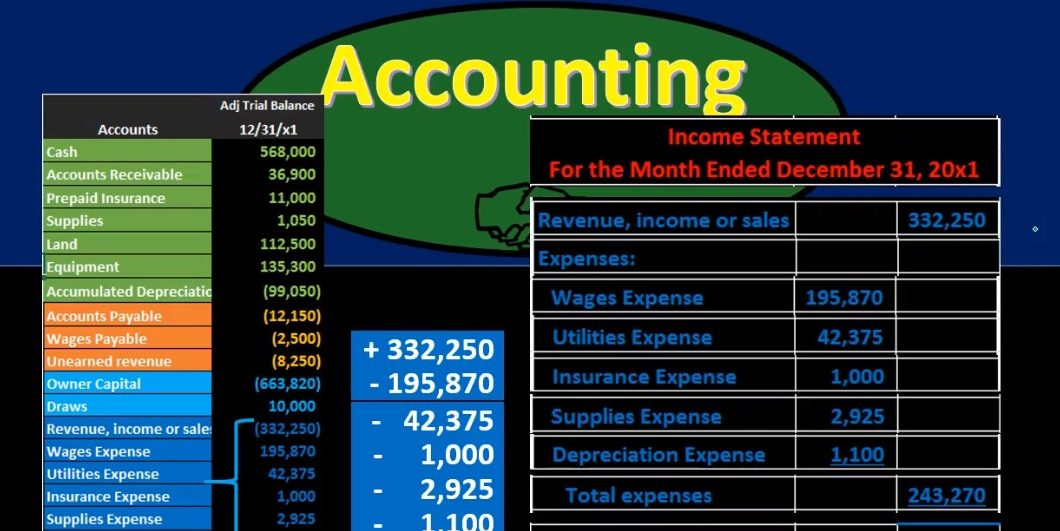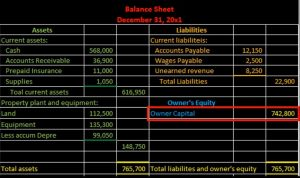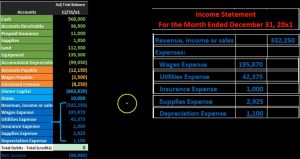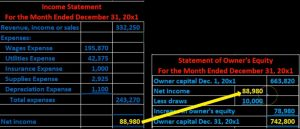## Income Statement from Trial Balance 16

Hello in this presentation we’re going to take a look at the creation of the income statement from the trial balance. First, we want to take a look at the trial balance and consider where the income statement accounts will be. When looking at the trial balance, it will be in order we have the assets in green, the liabilities in orange, the equity in light blue, and then the income statement accounts including revenue and expenses. That’s what we are concentrating here we’re looking at those income statement accounts. And that is what will be used in order to create the financial statements to create the income statement. Note that all the blue accounts represents the equity section. So the income statement really is going to be part of total equity. If we consider that on the balance sheet, then we’re really looking at a component of this capital account.

00:47

And it’s important to keep that in mind because we want to see where the income statement fits in with regards to the double entry accounting system with regards to the accounting equation, the accounting equation being represented here on the balance sheet. Where we have assets, we have liabilities, and we have the equity. So the income statement is going to give us detail. This is as of a point in time this equity account here, and we’re going to get more detail in terms of how that account got to where it is, by looking at some of the timing statements. The main one, of course, the income statement. So here’s going to be the income statement, we’re going to have revenue minus expenses. This is a single step income statement. So we’re just going to have groupings of all revenue accounts, minus the expense accounts, which will give us the net income, it’s important to look at how it’s going to be structured here, note that the revenue will be in the outer column. Just because we don’t have multiple revenue accounts. If we did, then we’d have a subcategory as we do here.01:46

But if we only have one account, we’re just going to pull up to the right. These do not mean debits and credits, these two columns, they just mean that we’re going to sub categorize anything on the left. And if it’s on the right then that’s going to be the sub category total. The total if it doesn’t have a subcategory, then we have the expenses which we have expenses colon, and then we indent all the expenses. And that’s going to give us an indication that these are all going to be grouped in the expenses, that subcategory. And we’re going to list those out on the inner column not because it’s a debit or credit, but because it’s going to be the listing of the accounts that we will then sum up in the outer section here. Then we have the total expenses, which we’re going to sum up in the outer section, and then we’re going to subtract those out to get net income. Note that we’re only doing thing with one columns at a time. So when we calculate net income, we’re not jumping back over here, we’re just subtracting the outer column, the revenue, minus the expenses.

02:43

Now we’re going to create the income statement from the trial balance. The income statement heading will typically have the company name, the statement of the income statement, and it’s important to note that it will have this for the month ended, in this case, December 31 2001. It’s important to note that Because that differs from what the balance sheet will have the balance sheet will just have one date, it’ll be as of a point in time, the income statement will have a range of dates, this case being the beginning of the month to the end of the month of December, we’re not going to do that with a range. Typically, we’re not going to show it by saying the beginning date and the ending date. What we’re going to do is have this terminology for the month ended December 31, in this case, 2000 x one. Now we’re going to go through and remember we’re picking up these accounts down here, we just want to find a home for all these accounts. Once we do, we should end up with net income.

03:36

So to do that, we’re going to say that first it’s going to be the revenue which is going to go from the top to the bottom, we’re going to pick up the revenue account. Note that this is represented as a credit with a bracket here, and we’re not going to show brackets up here, we’re just going to show a plus and minus format, because it is a revenue account and there’s only one of them. We’re going to put it in the right side here. So we’re going to put it on the right side. Not because debit or credit, but because it’s going to be subcategories, and there is no subcategory here, so it’s just going to be right on the right side, then we’re gonna have the expenses where we’re going to say expenses colon to represent that this is going to be a subcategory where we are going to group all of these expenses in the sub category under this expense heading, then we’ll pull over the expenses starting with the wages expense, which is pulling this number over here, we’re going to indent it a little bit, and we’re going to put that on the inner column to show that we’re going to group these together, and then sum them up to the outer column.

04:34

So remember, the inner column does not mean debit or credit, it means that these are going to be a sub category. Then we’re going to have the utilities expense, we’ll do the same thing we just pulled over the utilities expense, we put it in the inner column, which we will then subcategories out into the outer column. We have insurance expense, we’re just pulling this over here’s insurance expense. Pulling up in the Enter category. We have supplies expense that we’re just going to group over here as well. And finally, depreciation expense. Note, this is of course, a simplified or a pretty small type of income statement. If we had a lot of accounts here, it is very possible that we would have accounts within the income statement or the trial balance that we’d want to group together over here. For example, we might have multiple insurance type of accounts, multiple types of insurance that we want to group out so that we can see them in the distinct areas when we look at our books.05:29

But when we make the financial statements, the reader of the financial statements may only want to see one number, we may not want to have five insurance expense numbers that might not add value to the financial statements. So we might group them together in just one number when we pull them over here. Also note that the grouping or the ordering could change we have the ordering basically from the most expensive to the least, or the highest expense amount to the least. But that’s not typically the case. It’s not always see this is little out of order here. It’s not always is going to be that that way. But that’s one type of principle that we could use in terms of how do we want to order the expenses on the income statement. Once we’ve listed these out, we can add them up so that 195 870 plus 42 375 plus the 1000 plus the 2009 25, plus the 1100, will give us the total expenses of 243 270. We’re going to put total expenses here, we’re going to indent that once again, to show that this will be the total of the subcategory and put that in the outer column.

06:33

So note, we’re only adding up one column at a time. In this case, the this column here is what we’re adding up. We’re not adding up this and then jumping back to this column. We’re not doing things with two columns. We’re just doing things with this one column here. And then we’ll have our bottom line number, net income calculated as revenue, minus expenses. Once again, note that we’re only doing something with one column. Although these these two numbers are far apart. We’re only doing things with one one column at a time, we’re not jumping back over here and doing something with this column. And then back over here. That’s typically the format of all financial statements. And if you know that, it when you get to long financial statements, it can help you to break those down and not get overwhelmed to look at a lengthy financial statements.

07:18

If we’re going to see the trial balance over here, if we were to double check this, then in Excel, we could just highlight these and we could say, this is a credit which Excel sees as negative. And if I highlight all these, it’ll subtract out the debits, and that’ll give us the net income of 88 980. If we were to do that in a calculator, just to double check our net income number, we would take the revenue 330 to 250 minus the expense here of the 195 870 minus expense here 42 375 minus this expense of 1000 minus expense 2009 25 and minus 1100. That then if we add that up, or subtract that out, whatever the case may be, then we’re Get the 88 980. And that’ll give us a double check. And if we have this in Excel, it’s really easy for us to do these double checks as we go.

08:09

Now that we have the income statement, we can think about how it fits into the big picture. We’ve got the income statement here, how does it fit into the double entry accounting system? How does it tie into the other financial statements? Well, the income is going to give us that timing difference. And that net income number is going to be used to create the statement of owner’s equity. So the statement of owner’s equity is going to show us that equity section this is breaking out the equity section of the balance sheet gives us the beginning equity, and then there’s our net income.08:38

And then we’re going to subtract out draws, and that’ll give us our net change the net income minus the draws, and then we’re going to have our Indian equity which is going to be this beginning equity plus the change giving us that Indian equity. This is the amount that was on the balance sheet. So that’s how the income statement ties out to the balance sheet. That’s how the income statement ties out to the double entry accounting system through the account. accounting equation represented on the balance sheet as assets equal liabilities plus equity. This part is part of the calculation or one way we can break out the calculation of total equity here that will ultimately be on the balance sheet.